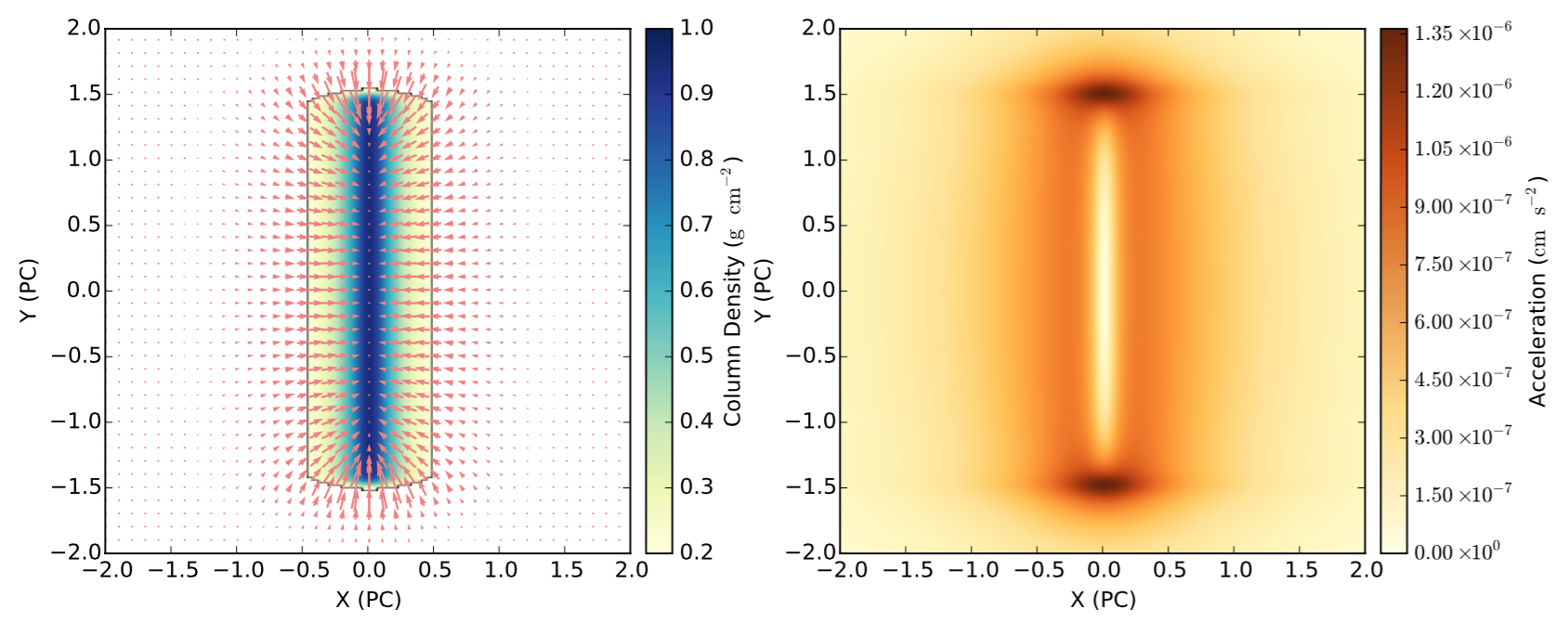# Mapping Gravity in Stellar Nurseries

Authors: G-X Li , A. Burkert, T. Megeath, and F. Wyrowski

First Author’s Institution: University Observatory Munich

Paper Status: Submitted to Astronomy and Astrophysics

Stars form when the densest parts of molecular clouds collapse under their own gravity. Molecular clouds are shaped by the interaction of gravity, turbulencemagnetic fields, and feedback from the energy and pressure exerted by stars. Turbulence is observed by measuring the statistical properties of the gas, magnetic fields can be observed using polarized light, and feedback can be identified by searching for protostellar outflows and other features in the cloud. But ultimately, gravitational collapse forms stars. Today’s paper proposes a method to map gravitational acceleration in molecular clouds.

The Method

Gravity comes from mass (thanks Isaac). To map the gravitational acceleration, we need a map of the mass in a molecular cloud. Molecular clouds are made up of gas and dust. The gas can be mapped using emission lines. The dust can be mapped by its effect on background starlight. The more dust in a cloud the dimmer and redder a background star appears. A map of the dust can be turned into the total column density by assuming an average factor between dust and gas mass. The gravitational acceleration is calculated for each pixel of a molecular cloud by adding the contributions from the mass in all other pixels.

The gravitational acceleration is a three-dimensional property, but observations only tell us the column density on the plane of the sky. We don’t know how the mass is distributed along the line of sight. The authors avoid this problem by assuming that all clouds have a uniform thickness of 0.3 pc. How bad is this assumption? It depends on the geometry of the cloud. A spherically symmetric cloud will have equal mass in front and behind the center of the cloud, contributing equal and opposite gravitational acceleration, so the assumption of uniform thickness is not bad. However, a filamentary cloud may be oriented at different angles to our line of sight, and could be much longer than it appears projected on the sky. These clouds appear more compact than they are, causing us to overestimate the acceleration within them. The authors stress that the acceleration maps are only accurate to an order of magnitude, and should be interpreted qualitatively.

Gravity On the Edge

Gravitational acceleration depends strongly on the shape of the cloud. Figure 1 shows the gravitational acceleration map of a simple disk. This cloud experiences the strongest gravitational acceleration in a ring around the edge of the disk. Figure 2 shows the acceleration in a simple filament. The strongest acceleration in this case is at the short ends of the cloud. Because of these edge effects, gravitational acceleration may enhance star formation in the edges of clouds.Figure 1. Uniform disk model of a molecular cloud. (Left) Cloud density is indicated in blue. Red arrows indicate the direction and magnitude of the gravitational acceleration in the cloud. (Right) The magnitude of acceleration is indicated in red. The acceleration is strongest in a ring around the edge of the disk. This effect can be seen in the Perseus molecular cloud (Figure 4).Figure 2. Filament model of a molecular cloud. The colors and arrows are the same as in Figure 1. In this case, the acceleration is strongest at the short ends of the filament. This effect can be seen in the Pipe Nebula (Figure 3).

Accelerating Star Formation

The authors make gravitational acceleration maps of several real  molecular clouds. The Pipe Nebula, shown in Figure 3, is a filamentary cloud. The acceleration is highest at the ends of the filament, like the model in Figure 2. The authors also show the positions of young stars in the Pipe Nebula. These young stars are mainly found in the areas of highest gravitational acceleration. The Perseus molecular cloud is shown in Figure 4. This cloud resembles the uniform disk model in Figure 1. Again, the gravitational acceleration is highest at the edges of this cloud. Young stars are found preferentially along the edges of the cloud. Both of these examples show that large acceleration may induce star formation. This could be because gas is flowing onto the clouds in these areas, enhancing the density enough to trigger collapse into stars.Figure 3. The Pipe Nebula. The colors and arrows are the same as in previous figures. The stars show the position of young stars in the cloud. The young stars tend to be found in areas of high acceleration, suggesting that star formation is triggered by gas accreting onto the cloud in these areas. Compare to the filament model in Figure 2.Figure 4. Perseus molecular cloud. The colors and arrows are the same as previous figures. The red contours show the region where dense cores of gas – the precursors of stars – are found. The star formation is preferentially occurring on the edge of the cloud, where the acceleration is highest. This effect is also seen in the simple uniform disk model in Figure 1.

The effects of gravitational acceleration may enhance star formation in areas of molecular clouds. But gravity is not the only force at work here. The effect of turbulence and magnetic fields need to be considered to judge the relative importance of gravity. The method presented here is complicated by theuncertainty in the three-dimensional structure of clouds, and the conversion between dust mass and total mass. By mapping the acceleration in simulated clouds, future studies may be able to quantify these uncertainties. Gravity is ultimately responsible for turning gas into stars, so understanding the role that gravity plays in molecular clouds is critical to a complete picture of star formation.